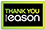# Mathematical methods for physics

PAPERBACK
From classical mechanics and classical electrodynamics to modern quantum mechanics many physical phenomena are formulated in terms of similar partial differential equations while boundary conditions determine the specifics of the problem. This 45th anniversary edition of the advanced book classic Mathematical Methods for Physics demonstrates how many physics problems resolve into similar inhomogeneous partial differential equations and the mathematical techniques for solving them. The text has three parts: Part I establishes solving the homogenous Laplace and Helmholtz equations in the three main coordinate systems, rectilinear, cylindrical, and spherical and develops the solution space for series solutions to the Sturm-Liouville equation, indicial relations, and the expansion of orthogonal functions including spherical harmonics and Fourier series, Bessel, and Spherical Bessel functions. Many examples with figures are provided including electrostatics, wave guides and resonant cavities, vibrations of membranes, heat flow, potential flow in fluids, and plane and spherical waves. In Part II the inhomogeneous equations are addressed where source terms are included for Poisson's equation, the wave equation, and the diffusion equation. Coverage includes many examples from averaging approaches for electrostatics and magnetostatics, from Green function solutions for time independent and time dependent problems, and from integral equation methods. In Part III complex variable techniques are presented for solving integral equations involving Cauchy Residue theory, contour methods, analytic continuation, and transforming the contour; for addressing dispersion relations; for revisiting special functions in the complex plane; and for transforms in the complex plane including Green's functions and Laplace transforms. Key Features: · Mathematical Methods for Physics creates a strong, solid anchor of learning and is useful for reference. · Lecture note style suitable for advanced undergraduate and graduate students to learn many techniques for solving partial differential equations with boundary conditions · Many examples across various subjects of physics in classical mechanics, classical electrodynamics, and quantum mechanics · Updated typesetting and layout for improved clarity This book, in lecture note style with updated layout and typesetting, is suitable for advanced undergraduate, graduate students, and as a reference for researchers. It has been edited and carefully updated by Gary Powell.
€68.59205 Reward Points
In stock online
Delivery in 2-3 working days

Any purchases for more than €10 are eligible for free delivery anywhere in the UK or Ireland!

From classical mechanics and classical electrodynamics to modern quantum mechanics many physical phenomena are formulated in terms of similar partial differential equations while boundary conditions determine the specifics of the problem. This 45th anniversary edition of the advanced book classic Mathematical Methods for Physics demonstrates how many physics problems resolve into similar inhomogeneous partial differential equations and the mathematical techniques for solving them. The text has three parts: Part I establishes solving the homogenous Laplace and Helmholtz equations in the three main coordinate systems, rectilinear, cylindrical, and spherical and develops the solution space for series solutions to the Sturm-Liouville equation, indicial relations, and the expansion of orthogonal functions including spherical harmonics and Fourier series, Bessel, and Spherical Bessel functions. Many examples with figures are provided including electrostatics, wave guides and resonant cavities, vibrations of membranes, heat flow, potential flow in fluids, and plane and spherical waves. In Part II the inhomogeneous equations are addressed where source terms are included for Poisson's equation, the wave equation, and the diffusion equation. Coverage includes many examples from averaging approaches for electrostatics and magnetostatics, from Green function solutions for time independent and time dependent problems, and from integral equation methods. In Part III complex variable techniques are presented for solving integral equations involving Cauchy Residue theory, contour methods, analytic continuation, and transforming the contour; for addressing dispersion relations; for revisiting special functions in the complex plane; and for transforms in the complex plane including Green's functions and Laplace transforms. Key Features: · Mathematical Methods for Physics creates a strong, solid anchor of learning and is useful for reference. · Lecture note style suitable for advanced undergraduate and graduate students to learn many techniques for solving partial differential equations with boundary conditions · Many examples across various subjects of physics in classical mechanics, classical electrodynamics, and quantum mechanics · Updated typesetting and layout for improved clarity This book, in lecture note style with updated layout and typesetting, is suitable for advanced undergraduate, graduate students, and as a reference for researchers. It has been edited and carefully updated by Gary Powell.
Quantity:
In stock online
Delivery in 2-3 working days205 Reward Points

Any purchases for more than €10 are eligible for free delivery anywhere in the UK or Ireland!

€68.59
In stock online
Delivery in 2-3 working days